# Deep Learning-Based Forecast and Warning of Floods in Klang River, Malaysia

Deep Learning-Based Forecast and Warning of Floods in Klang River, Malaysia

Amrul Faruq Hudan Pandu Arsa Shamsul Faisal Mohd Hussein  Che Munira Che Razali Aminaton Marto  Shahrum Shah Abdullah

Malaysia-Japan International Institute of Technology (MJIIT), Universiti Teknologi Malaysia, Kuala Lumpur 54100, Malaysia

Department of Electrical Engineering, Faculty of Engineering, Universitas Muhammadiyah Malang (UMM), Malang 65144, Indonesia

Department of Geomatics Engineering, Faculty of Civil, Environmental, and Geo Engineering, Institute of Technology Sepuluh Nopember (ITS), Surabaya 60111, Indonesia

Corresponding Author Email:
shahrum@utm.my
Page:
365-370
|
DOI:
https://doi.org/10.18280/isi.250311
7 March 2020
|
Accepted:
4 May 2020
|
Published:
30 June 2020
| Citation

OPEN ACCESS

Abstract:

Long short-term memory (LSTM) networks are state of the art technique for time-series sequence learning. They are less commonly applied to the hydrological engineering area especially for river water level time-series data for flood warning and forecasting systems. This paper examines an LSTM network for forecasting the river water level in Klang river basin, Malaysia. The river water level contains of two features dimension and one time-series observed data, in this study, prediction responses for river water level data using a trained recurrent neural network and update the network state function is applied. The radial basis function neural network (RBFNN) in order to get comparison of the generalization solving problem also performed. The performance indicates with the root mean square error, RMSE 0.0253 and coefficient of determination value, R2 0.9815 are closely accurate when updating the network state compared with the RBFNN results. These results verified that the LSTM network with specified training set options is a promising alternative technique to the solution of flood modelling and forecasting problems.

Keywords:

flood forecasting, early warning system, deep learning, machine learning

1. Introduction

Urban floods are considered one of the wide-scale devastating natural disasters affected globally, and improved flood forecasting model is essential for better flood warning and management system. It has become an issue of great importance recently due to extensive damage to human life, properties, and lead to significant socioeconomic problems caused by floods. Flood forecasting system plays a crucial role in planning and regulating the disaster risk reduction and management (DRRM). The Sendai frameworks 2015-2030, highlighted ‘investing in disaster risk reduction for resilience’ and ‘enhancing disaster risk preparedness for effective response’ are respectively for priority number three and four regarding the DRRM .

Research on flood forecasting models has a long tradition in the hydrological engineering area. However, the determination of improved flood modelling and forecasting is technically challenging. As reported in numerous published studies, river water level forecasting for flood management is a difficult task and highly dynamic to model. Recently, researchers have shown an increased interest in river flood prediction and modelling, namely, data-driven methods. The types of models use the generalized relationship between input and output variable datasets without requiring the physical mechanism behind the process of the model. In which, the model is constructed based on historical data.

Neural network models, especially as data-driven approaches, are developed through training the network to demonstrate the relationships and processes that are inherent within the data. Research on flood water level forecasting has been successfully taken by the authors [2, 3]. The works are growing advance in exploring more suitable flood forecasting model. Reviewing some published research works, by using the Artificial Neural Networks (ANNs) model, Elsafi  has demonstrated real-time flood forecasting in the case of River Nile at Dongola Station. Reference  explored the applicability of a deep learning approach for predicting hourly air temperature using real sensor data of Northwestern Nevada region. The results indicate that stacked de-noising auto-encoder (SDAE) as deep learning approach performs better rather than standard neural networks model. Moreover, as a part of machine learning methods, ANNs is the most popular Artificial Intelligent (AI)-based technique in flood forecasting .

The continued advancement of AI methods, in recent years has seen a growing trend towards deep learning techniques and their application in time-series prediction. Deep learning is being studied in many types of problems such as image processing, speech recognition, and natural language processing. In the field of forecasting, a recent experiment has been reported the successful use of deep learning in various fields, respectively for power load and probability density forecasting , traffic flow forecasting , and rainfall forecasting . As it developed that deep learning can be quite promising, reported better results than the traditional ANN model . Two of the most growing research points in deep learning are enhancing computer vision using convolutional neural networks (CNN) and modelling time-series or sequential data through a recurrent neural network (RNN) .

More modern RNN architectures were proposed since the late in the 1990s, and one of the successful networks is the long short-term memory (LSTM) [11, 12]. Although studies have recognized LSTM in solving time-series prediction , to date, few published research works have explored the use of LSTM as deep learning approach in hydrological engineering problems, especially for flood forecasting. Thus, it is a chance to employs LSTM network to predict river water levels for flood forecasting and warning system. This study is to build real-time data-driven models that enable to simulate and predict river water level from historical data using LSTM network. The long short-term memory network is a type of recurrent neural network used in deep learning due to large architecture can be successfully trained.

This paper organized as follows; in Section 2, the proposed LSTM network model, RBFNN model, a case study as flood forecasting point (FFP), and set of datasets are presented. Including performance evaluation formula to measure the forecasted model performance. Result and discussion of the application of deep learning LSTM network for time-series flood forecasting problems is described in Section 3. Followed by section 4, summarizes of the conclusion of this work. Finally, research challenges and future work direction also addressed in this paper.

2. Methodology

2.1 Deep learning long short-term memory network

A simple and effective model for flood forecasting is designed in this study. The river water level collected from the Klang River at Sulaiman Bridge, Malaysia, is used as input dataset. The total available data was used to train and test using the LSTM model. The main feature of the LSTM network is the hidden layer called memory cells. LSTM networks consist of an input layer, one or more memory cells, and an output layer. The number of neurons in the input layer is equal to the number of explicative variables [11, 12]. Each of the memory cells has three gates maintaining and adjusting its cell state St: a forget gate (ft), an input gate (it), and output gate (Ot), Figure 1 shows the LSTM architecture memory cell.

The state of the layer consists of the hidden state (also known as the output state) and the cell state. The hidden state at time step t contains the output of the LSTM layer for this step. The cell state contains information learned from the previous time steps. At each time step, the layer adds information to or remove information from the cell state. The layer controls these updates using gates. At every time-step t, each of the three gates is presented with the input xt (one element of the input sequence) as well as the output ht-1 of the memory cells at the previous time-step t-1. According to the references [11, 12] and , hereby, the gates act as filters, each different fulfilling purposes:

• The forget gate defines what information is removed from the cell state
• The input gate specifies what information is added to the cell state
• The output gate specifies what information from the cell state is used,

and the sequential update formulas can be referred to Hu et al. . The specified topology of the proposed LSTM networks is according to the neural network deep learning toolbox provided by MATLAB® function can be seen in Table 1, to predict time steps one at a time and update the network state at each prediction.

In order to train the LSTM model with the water level element data, the typical working mechanism of the LSTM can be seen in Figure 1, according to Fischer and Krauss . The Klang river water level data measured every fifteen minutes indicated as a vector xt and the multiple vectors into time series data. This time series water level xt setting up as input to the LSTM network and the output of the network is prediction results. The fully connected layers are connected to the LSTM network. The number of features and response are respectively indicated the number of inputs and output.

## 11.1.png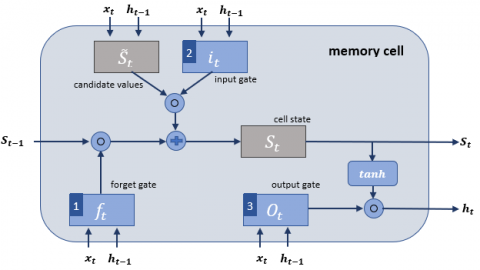Figure 1. The LSTM network architecture memory cell according to Fischer and Krauss 

Table 1. Parameters setting used in LSTM network

 Variables Value Number of features / responses 2 / 1 Time step 1 LSTM layer hidden units 100 Network type Fully connected Training dataset 10000 Validation test 2000 Training epochs 100 Gradient Threshold 1 Initial learn rate 0.01 Learn rate drop period 125 Learn rate drop factor 0.2 State and Gate activation function tanh and sigmoid

Firstly, removed information from its previous cell states St-1  would determines by the LSTM layer. Therefore, the activation values ftof the forget gates at timestep t are computed based on the current input and output, xt and ht-1 of the memory cells at the previous timestep, t-1, and the bias terms of the forget gates, bf. The sigmoid function then scales all activation values into the range of 0 and 1, respectively as completely forgot and completely remembered.

In the second step, the LSTM layer determines the information would be added to the network's cell states, St Computes the candidate values $\widetilde{S}_{t}$ and then the activation values of the input gates.

In the third step, the new cell states St are calculated based on the results of the previous two steps with denoting the elementwise product. Finally, the output ht of the memory cells unit is obtained [11, 14].

## 11.2.png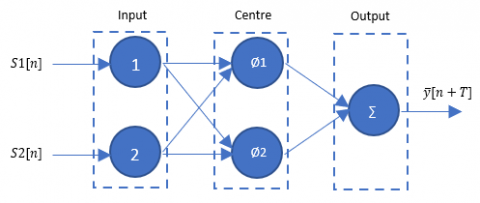Figure 2. Architecture of RBFNN used in this study

2.2 Radial basis function neural network

The RBF architecture and their input used in this study as shown in Figure 2. The network consists of three layers: an input layer, a hidden layer, and an output layer. Here, R denotes the number of inputs. In which, two upstream-rivers water level and one flood water level at FFP. While Q the number of output, predicted water level of the Klang River at time n+T, and T is 0 hour as known as real-time evaluation. Eq. (1) is used to calculate the output of the RBFNN. For Q=1, the output of the RBFNN in Figure 2 is:

$\eta(x, w)=\sum_{k=1}^{S 1} w_{1 k} \phi\left(\left\|x-c_{k}\right\|\right)_{2}$  (1)

In which $x \in \mathcal{R}^{R x 1}$ is an input vector,$\emptyset(.)$ is a basis function, $\|\cdot\|_{2}$ denotes the Euclidean norm, w1k are the weights in the output layer, S1 is the number of neurons (and centers) in the hidden layer and $C_{k} \in \mathcal{R}^{R x 1}$ are the RBF centers in the input vector space.

The basis function Ck is set equal to input vector from training data sets, 2. Then 10 number of spreads is used in the training process. A large spread implies a lot of neurons required to fit a fast-changing function, where a small spread is means less neuron to fit smooth function and the network may not generalized well.

2.3 Data set size

The usefulness of the approached method is evaluated using a case study for the Klang River, Kuala Lumpur, Malaysia. The location of the selected station is shown in Figure 3 with the red triangle detection. The Klang River was chosen as a flood forecasting point based on the department of irrigation water level at Sulaiman Bridge. The total catchment area up to the station is approximately 466 km2 . The Klang river has total stream length of about 1200 km2 at the mount of Port Klang, Selangor. The main river has headwaters in the mountain area to the north-east of Kuala Lumpur, where the maximum elevation rises to the above of 1400 m . About 76K with fifteen minutes basis time-series observations dataset provided by department of irrigation and drainage (DID) system Malaysia recorded in November 2011-December 2013. And measured data from January – May 2013 were used as historical input to the LSTM network. The five months data recorded about 10000 datasets examined as training and 2000 data used as validation test, respectively.

## 11.3.png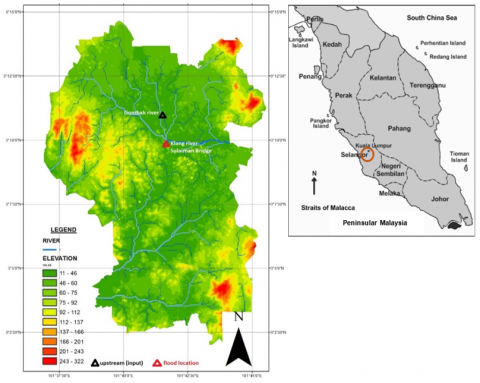Figure 3. Selected study area, Klang River basin at Sulaiman Bridge, Kuala Lumpur, as flood forecasting point

In order to demonstrate the efficiency of the LSTM network model, in this study, performance is assessed by using the root mean square error, RMSE. It is formulated in Eq. (2). While R2 in Eq. (3) is the coefficient of determination, value describes the proportion of the variance in the observed dataset that can be explained by the model.

$R M S E=\sqrt{\frac{1}{n} \sum_{i=1}^{n}\left|y-y^{\prime}\right|^{2}}$  (2)

$R^{2}=\frac{\sum_{i=1}^{n}\left(\cdot y^{\prime}-\bar{y}\right)^{2}}{\sum_{i=1}^{n}(\cdot y-\bar{y})^{2}}$  (3)

in which, n is the number of data points, y’ represents the forecasted value of river water level, y is the observed river water level at the time i, and $\bar{y}$ is the average value of the actual or observed records. In this study, Matlab and its environment were used to be the platform for programming and simulation tool in establishing the developed model.

3. Result and Discussion

The simulated model is done using neural network deep learning MATLAB® function to train an LSTM network for deep learning. For a better fit and to prevent the training from diverging, standardized the training data is applied to have zero mean and unit of variance as it applied in previous study . The normalized data obtained from the difference between training data and the mean of training data divided by the standard deviation value of training data.  At prediction time, the normalized test data using the same parameters as the training data. The normalized water level data can be illustrated in Figure 4. In this study, the network state was initialized at first using 10000 training data set and then simulated the next prediction of 2000 validation test data using the last time step of the training response, continued by looping over the remaining predictions and input the previous prediction to the LSTM network.

The experiment result of 2000 validation data sets can be referred in Figure 5 with the red line distribution values. Quantitatively, with forecasted and observed values both are unstandardized to their original scale. From Figure 5, it showed that the forecasted red line distribution value quite follows the validated pattern from the actual value after it’s unstandardized. The result was shown in original stage river water level. The result shown in Figure 6 seems that the LSTM network provides a considerable accurate in terms of coefficient determination value. Although LSTM has difficulties in explaining the detailed peak values, the network is adequate to follow the dominant trends which is very important for flood warning analysis . The RMSE and the coefficient of determination, Rwere calculated to evaluate model performance. These two evaluated performances were calculated from the unstandardized prediction results. The results showed that the RMSE and Rrespectively of 0.0253 and 0.9815 for LSTM model, and 0.06 and 0.9155 for RBFNN, which showed quit precision. In this case, the LSTM model outperformed the RBFNN .

The LSTM network generally reflected observed river water level. Despite the fact that the LSTM networks has complexity and highly non-linear model, the RBFNN is capable of providing good prediction with the network configuration promises simply model and smaller than LSTM . In addition, comparing with the peak water level simulation, the RBFNN result have accurately predicted as shown in Table 2.

## 11.4.png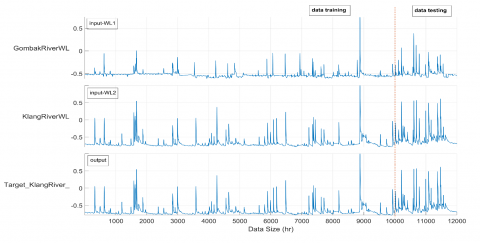Figure 4. Hourly River Klang water level standardized data

Table 2. Klang river water level indicator for flood warning system

 Normal Level (Green) Alert Level (Yellow) Warning Level (Orange) Danger Level (Red) Highest Level Average Level Actual LSTM RBFNN Actual LSTM RBFNN 23.00 M 27.00 M 28.25 M 29.50 M 26.46 M 26.25 M 26.45 M 22.80 M 22.78 M 22.78 M

## 11.6.pngFigure 5. Hourly River Klang water level forecasting and observed data after unstandardized

## 11.61.png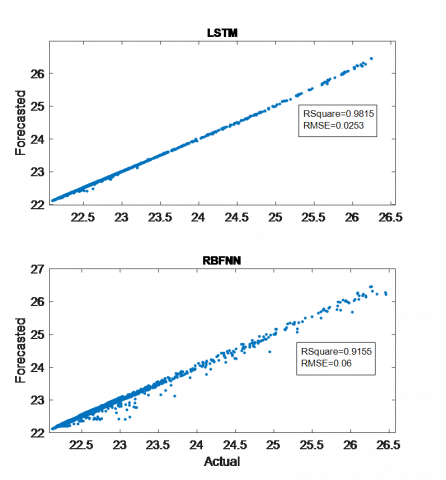Figure 6. Scatter-gram distribution of the observed and forecasted river water level of RBFNN and LSTM

After the quantitative analysis of both LSTM and RBFNN models, the observed and simulated water level distribution value also scattered. The LSTM model has a high value indicating that the model could well reflect the relationship between observed and simulated results. From Figure 6, the data is scattered relatively closer to the LSTM model. It is clearly shown that LSTM model is a good correlation with the observed water level data.

3.1 Flood forecasting and warning system

In connection with disaster risk management and flood warning system, according to DID [20, 21] for river water level data-above mean sea level, it can be classified as three main categories, including “alert level”, “warning level”, and “danger level”. The alert level for Klang River, in Sulaiman Bridge is 27.00 meters, while for warning level is 28.25 meters, and danger level is 29.50 meters – above mean sea levels . According to DID, the maximum value is about 27.314 meters categorized as “alert level” at that time.

It indicates when the river water level more than the maximum observed value, can be assumed flood will occur in that particular area since it could be reached at a danger level. As an early warning system this flood forecasting model using deep learning technique could be more effective tools to detect flood water level at the certain levels.

4. Conclusion

This paper set out to develop a simulated model for river water levels using the LSTM and RBFNN network. The study has examined the concept of RNN as deep learning LSTM cell. The LSTM model not only takes full advantage of the current data characteristics but also uses its gate structure to decide whether to remember or forget the previous feature. With the progress of AI techniques, the deep learning method of long short-term memory network model could be better used in the hydrological engineering problems, due to the LSTM is very effective in modelling large time-series data. In this paper, the input data set was considered from two upstream to forecast the river water level of the next hours for flood warning system. The result verified that the LSTM network with specified training set options is a promising alternative technique to the solution of flood modeling and forecasting problems. The overall performance indicates with the root mean square error, RMSE 0.0253 and R2 value 0.9815 are closely accurate when updating the network state compared with kind of ANN model, which is RBFNN. Nevertheless, both LSTM and RBFNN networks performed adequately to forecast the river water level in order to meet flood warning levels.

For further research study, employing more input variables including weather forecast, rainfall data, and streamflow data can be addressed in order to get the relationship impact of the improved flood forecasting performance. Furthermore, the enhancement of machine learning algorithm in advance AI-based methods will be more challenging to develop robustness of such time series forecasting problems.

Acknowledgemnet

The authors would like to acknowledge the Malaysia-Japan International Institute of Technology, Universiti Teknologi Malaysia, for supporting and facilitating this work. As well as the department of irrigation and drainage (DID) Malaysia for the research materials supplied. The first author thanks to the Universitas Muhammadiyah Malang for giving a chance to undertaking this study.

References

 UNISDR, Sendai Framework for Disaster Risk Reduction 2015-2030, 2015.

 Faruq, A., Abdullah, S.S., Marto, A., Bakar, M.A., Hussein, S.F.M., Razali, C.M.C. (2019). The use of radial basis function and non-linear autoregressive exogenous neural networks to forecast multi-step ahead of time flood water level. International Journal of Advances in Intelligent Informatics, 5(1): 1-10. https://doi.org/10.26555/ijain.v5i1.280

 Abu Bakar, M.A., Abdul Aziz, F.A., Mohd Hussein, S.F., Abdullah, S.S., Ahmad, F. (2017). Flood water level modeling and prediction using radial basis function neural network: Case study kedah. Communications in Computer and Information Science, 751: 225-234. https://doi.org/10.1007/978-981-10-6463-0_20

 Elsafi, S.H. (2014). Artificial Neural Networks (ANNs) for flood forecasting at Dongola Station in the River Nile, Sudan. Alexandria Engineering Journal, 53(3): 655-662. https://doi.org/10.1016/j.aej.2014.06.010

 Hossain, M., Rekabdar, B., Louis, S.J., Dascalu, S. (2015). Forecasting the weather of nevada: a deep learning approach. 2015 International Joint Conference on Neural Networks (IJCNN), Killarney, Ireland. https://doi.org/10.1109/IJCNN.2015.7280812

 Suliman, A., Nazri, N., Othman, M., Malek, M.A., Ku-Mahamud, K.R. (2013). Artificial neural network and support vector machine in flood forecasting: A review. Proceedings of the 4th International Conference on Computing and Informatics, ICOCI 2013, Sarawak, Malaysia, pp. 327-332.

 Guo, Z., Zhou, K., Zhang, X., Yang, S. (2018). A deep learning model for short-term power load and probability density forecasting. Energy, 160: 1186-1200. https://doi.org/10.1016/j.energy.2018.07.090

 Qu, L., Li, W., Li, W., Ma, D., Wang, Y. (2019). Daily long-term traffic flow forecasting based on a deep neural network. Expert Systems with Applications, 121: 304-312. https://doi.org/10.1016/j.eswa.2018.12.031

 He, X., Luo, J., Zuo, G., Xie, J. (2019). Daily runoff forecasting using a hybrid model based on variational mode decomposition and deep neural networks. Water Resources Management, 33: 1571-1590. https://doi.org/10.1007/s11269-019-2183-x

 Cai, M., Pipattanasomporn, M., Rahman, S. (2019). Day-ahead building-level load forecasts using deep learning vs. traditional time-series techniques. Applied Energy, 236: 1078-1088. https://doi.org/10.1016/j.apenergy.2018.12.042

 Hu, C., Wu, Q., Li, H., Jian, S., Li, N., Lou, Z. (2018). Deep learning with a long short-term memory networks approach for rainfall-runoff simulation. Water, 10(11): 1-16. https://doi.org/10.3390/w10111543

 Hochreiter, S., Schmidhuber, J. (1997). Long short-term memory. Neural Computation, 9(8): 1735-1780. https://doi.org/10.1162/neco.1997.9.8.1735

 Gensler, A., Henze, J., Sick, B., Raabe, N. (2016). Deep learning for solar power forecasting – an approach using autoencoder and LSTM neural networks. 2016 IEEE International Conference on Systems, Man, and Cybernetics (SMC), Budapest, Hungary, https://doi.org/10.1109/SMC.2016.7844673

 Fischer, T., Krauss, C. (2018). Deep learning with long short-term memory networks for financial market predictions. European Journal of Operational Research, 270(2): 654-669. https://doi.org/10.1016/j.ejor.2017.11.054

 Goh, Y.C., Zainol, Z., Mat Amin, M.Z. (2016). Assessment of future water availability under the changing climate: case study of Klang River Basin, Malaysia. International Journal of River Basin Management, 14(1): 65-73. https://doi.org/10.1080/15715124.2015.1068178

 Wardah, T., Abu Bakar, S.H., Bardossy, A., Maznorizan, M. (2008). Use of geostationary meteorological satellite images in convective rain estimation for flash-flood forecasting. Journal of Hydrology, 356(3-4): 283-298. https://doi.org/10.1016/j.jhydrol.2008.04.015

 Sahoo, B.B., Jha, R., Singh, A., Kumar, D. (2019). Long short-term memory (LSTM) recurrent neural network for low-flow hydrological time series forecasting. Acta Geophysica, 67: 1471-1481. https://doi.org/10.1007/s11600-019-00330-1

 Kim, H.U., Bae, T.S. (2017). Preliminary study of deep learning-based precipitation prediction. Journal of the Korean Society Surveying Geodesy Photogrammetry Cartography, 35(5): 423-430. https://doi.org/10.7848/ksgpc.2017.35.5.423

 Chang, F.J., Liang, J.M., Chen, Y.C. (2001). Flood forecasting using radial basis function neural networks. IEEE Transactions on Systems, Man, and Cybernetics, Part C (Applications and Reviews), 31(4): 530-535. https://doi.org/10.1109/5326.983936

 Azad, W.H., Weisgerber, A., Ahmad F., Fauzi, F., Ghazali, N.H.M. (2019). National flood forecasting and warning system of Malaysia: Automated forecasting for the east. Hydrol. Water Resour. Div. Dep. Irrig. Drain. (JPS), Malaysia.

 Sulaiman, A.H. (2019). Flood management in Malaysia. Department of Irrigation and Drainage Systems. http://water.gov.my/, accessed on Mar. 28, 2019.

 DID Malaysia. On-line river level data - above mean sea level. Department of Irrigation and Drainage, Malaysia. http://infobanjir.water.gov.my/, accessed on Dec. 07, 2019.